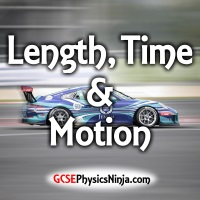# Length, Time & MotionWelcome to the GCSE Physics Ninja Length, Time & Motion course.

These video lessons are based on question-and-answer flashcards. You can either make your own or purchase the printable flashcards from the shop.

## What will I learn in this course?"

When you have completed this course, you will be able to...

• Become familiar with the quantities and units used to measure length, time and motion.
• Measure short time intervals with reasonable accuracy.
• Use a micrometer screw gauge to measure very small lengths.
• Measure and calculate the density of a substance.
• Understand how to interpret distance-time graphs and velocity-time graphs.
• Calculate distance travelled, speed and acceleration of an object using a graph, or an equation.
• Explain how objects can move in circular motion.
• Perform calculations on simple pendulums.
• Understand what vectors are, and how to find a resultant vector graphically.